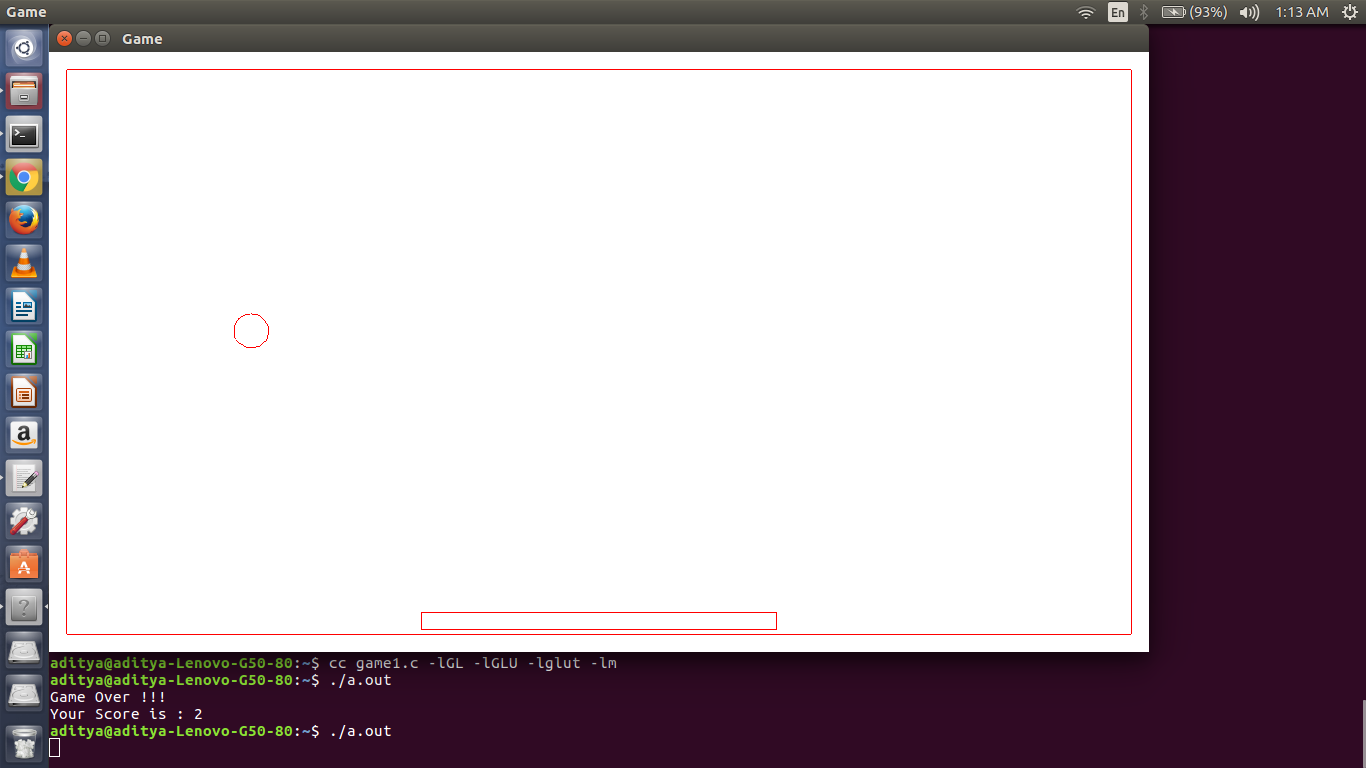# OpenGL program for Simple Ball Game

• Difficulty Level : Expert
• Last Updated : 15 Jun, 2017

Prerequisite – OpenGL

OpenGL is a cross-language, cross-platform API for rendering 2D and 3D Vector Graphics. Using this, we can make a lot of design as well as animations. Below is a simple Game made using OpenGL.

Take a step-up from those "Hello World" programs. Learn to implement data structures like Heap, Stacks, Linked List and many more! Check out our Data Structures in C course to start learning today.

Description : In this, a ball is moving starting from middle and goes to up-left in starting. But, then it collides with wall and changes direction but speed remain same. Consequently, it comes to downward direction where it should be catched on a rectangle (which is capable of moving in horizontal direction) in order to continue.

For working on Ubuntu operating system:

```gcc filename.c -lGL -lGLU -lglut -lm
where filename.c is the name of the file
with which this program is saved.
```

## Recommended: Please try your approach on {IDE} first, before moving on to the solution.

 `// C program to illustrate OpenGL game``#include``#include``#include``#define pi 3.142857`` ` `// Global Declaration``// c and d tracks the number of time 'b' and 'n' pressed respectively``// left and right indicates leftmost and rightmost index of movable rectangle``int` `c = 0, d = 0, left = 0, right = 0;``int` `m = 0, j = 1, flag1 = 0, l = 1, flag2 = 0, n = 0, score = 0, count = 1;`` ` `// Initialization function``void` `myInit (``void``)``{``    ``// Reset background color with white (since all three argument is 1.0)``    ``glClearColor(1.0, 1.0, 1.0, 0.0);`` ` `    ``// Set picture color to red (in RGB model)``    ``// as only argument corresponding to R (Red) is 1.0 and rest are 0.0``    ``glColor3f(1.0f, 0.0f, 0.0f);`` ` `    ``// Set width of point to one unit``    ``glPointSize(1.0);``    ``glMatrixMode(GL_PROJECTION);``    ``glLoadIdentity();`` ` `    ``// Set window size in X- and Y- direction``    ``gluOrtho2D(-620.0, 620.0, -340.0, 340.0);``}`` ` `// keyboard function : it gets active when button pressed``void` `keyboard(unsigned ``char` `key, ``int` `x, ``int` `y)``{``    ``left = -200 + 200 * (d - c);``    ``right = 200 + 200 * (d - c);`` ` `    ``// if leftmost index of window is hit``    ``// then rectangle will not move to left on furthure pressing of b``    ``// only it will move to right on pressing n``    ``if` `(left == -600)``    ``{``        ``// '110' -> Ascii value of 'n'``        ``// so d is incremented when n is pressed``        ``if` `(key == 110)``            ``d++;``    ``}``    ``// if rightmost index of window is hit``    ``// then rectangle will not move to right on furthure pressing of n``    ``// only it will move to left on pressing b``    ``else` `if` `(right == 600)``    ``{``        ``// '98' -> Ascii value of 'b'``        ``// so c is incremented when b is pressed``        ``if` `(key == 98)``            ``c++;``    ``}``    ``// when rectangle is in middle, then it will move into both``    ``// direction depending upon pressed key``    ``else``    ``{``        ``if` `(key == 98)``            ``c++;    ``        ``if` `(key == 110)``            ``d++;``    ``}``    ``glutPostRedisplay();``}`` ` `void` `myDisplay(``void``)``{``    ``// x and y keeps point on circumference of circle``    ``int` `x, y, k;``    ``// outer 'for loop' is to for making motion in ball``    ``for` `(k = 0; k <= 400; k += 5)``    ``{    ``        ``glClear(GL_COLOR_BUFFER_BIT);``        ``glBegin(GL_LINE_STRIP);``        ``// i keeps track of angle``        ``float` `i = 0;``        ``// change in m denotes motion in vertical direction and``        ``// change in n denotes motion in horizontal direction``        ``m = m + 6;``        ``n = n + 4;``        ``// drawing of circle centre at (0, 12) iterated up to 2*pi, i.e., 360 degree``        ``while` `(i <= 2 * pi)``        ``{``            ``y = 12 + 20 * ``cos``(i);``            ``x = 20 * ``sin``(i);``            ``i = i + 0.1;``            ``// flag1 is 0 to show motion in upward direction and is 1 for downward direction``            ``if` `(m == 288 && flag1 == 0)``            ``{``                ``j = -1;``                ``m = -288;``                ``flag1 = 1;``                ``score++;``            ``}``            ``if` `(m == 288 && flag1 == 1)``            ``{``                ``j = 1;``                ``m = -288;``                ``flag1 = 0;``            ``}``            ``// flag2 is 0 to show motion in rightward direction and is 1 for leftward direction``            ``if` `(n == 580 && flag2 == 0)``            ``{``                ``l = -1;``                ``n = -580;``                ``flag2 = 1;``            ``}``            ``if` `(n == 580 && flag2 == 1)``            ``{``                ``l = 1;``                ``n = -580;``                ``flag2 = 0;``            ``}``            ``// equation for desired motion of ball``            ``glVertex2i((x - l * n), (y - j * m));``        ``}``        ``glEnd();`` ` `        ``// these four points draws outer rectangle which determines window``        ``glBegin(GL_LINE_LOOP);``            ``glVertex2i(-600, -320);``            ``glVertex2i(-600, 320);``            ``glVertex2i(600, 320);``            ``glVertex2i(600, -320);``        ``glEnd();`` ` `        ``// these four points draws smaller rectangle which is for catching ball``        ``glBegin(GL_LINE_LOOP);``        ``left = -200 + 200 * (d - c);``        ``right = 200 + 200 * (d - c);``            ``glVertex2i(left, -315);``            ``glVertex2i(left, -295);``            ``glVertex2i(right, -295);``            ``glVertex2i(right, -315);``        ``glEnd();`` ` `        ``// following condition checks if falling ball is catched on rectangle or not``        ``if` `((j * m) == 276)``        ``{``            ``if` `((left > ((-1 * l * n) + 20)) || (right < (-1 * l * n) - 20))``            ``{``                ``printf``(``"Game Over !!!\nYour Score is :\t%d\n"``, score);``                ``exit``(0);``            ``}``        ``}``        ``glutSwapBuffers();``    ``}``}`` ` ` ` `// Driver Program``int` `main (``int` `argc, ``char``** argv)``{``    ``glutInit(&argc, argv);``     ` `    ``glutInitDisplayMode(GLUT_DOUBLE | GLUT_RGBA);``    ``// Declares window size``    ``glutInitWindowSize(1100, 600);``     ` `    ``// Declares window position which is (0, 0)``    ``// means lower left corner will indicate position (0, 0)``    ``glutInitWindowPosition(0, 0);`` ` `    ``// Name to window``    ``glutCreateWindow(``"Game"``);`` ` `    ``// keyboard function``    ``glutKeyboardFunc(keyboard);``    ``// Call to myInit()``    ``myInit();``    ``glutDisplayFunc(myDisplay);``    ``glutMainLoop();``}`

Below is the screenshot of the animation. This screenshot is of one instance. In actual, ball will move in different direction which should be catched on the smaller rectangle. Rectangle can be moved to left by pressing lowercase b and towards right by lowercase n. However, this can be changed by changing the code in keyboard function where ASCII value of key can be changed.